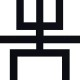# Legoplots in R (3D barplots in R)

I previously mentioned these, but I thought about it some more and went ahead and wrote an R implementation.  With very little work you could rejig this code to display all kinds of 3D bar plots; I’ve commented the code thoroughly so it should be easy to follow.  Immediately below is an example plot and below that is a gallery comparing an original plot by the Broad Institute with two versions produced by my code.  I wouldn’t want to slavishly copy, so I’ve added an optional transparency effect that is both useful and attractive.

### Arguments

• `z` : a named vector of length 96 representing each of the 6 possible mutations in 16 different sequence contexts.  Note that the names are hard-coded and can be found in the code below.  Values represent the height of each column
• `alpha `: transparency of column sides.  Top is always opaque.  Default is 1 (opaque)
• `scalexy` : value to scale the area of all columns.  Small values lead to skinny columns, larger values lead to fatter columns.  Default is 10
• `scalez` : value to scale the height of all columns; see notes below.  Default is 1
• `gap` : a value determining the space between each column.  Default is 0.2

### Things to note

• It was essential to include the `lit=FALSE` argument when drawing surfaces, as the default lighting makes everything look very shiny and pretty ugly.  I also had to change the field of view (FOV) to 0, as the default value gives a slightly wall-eyed view.
• The scalexy argument scales both the “fatness” of the columns and the gaps between them.
• Scaling z using the scalez argument is useful if your data is very flat and you want to emphasize it.  It will have knock-on effects on the scale that appears at the side, so be careful.  If you’re going to transform your data, it’s probably best to do so before putting it into this function.
• The alpha transparency I added to the column sides is very useful for viewing columns that are obstructed by other columns and has the added benefit of looking pretty cool.
• I haven’t included the wireframe axis background, I leave that as an exercise for the reader, or maybe I’ll add it later.

Code is available below and example data is included if you check out my GitHub here.

```## This script creates a "legoplot" similar to those produced by the Broad Institute
## The plot shows the relative abundance of each of the 6 possible mutations in the
## 16 sequence contexts

library(rgl)

#### START OF FUNCTIONS

## Functions modified from the "demo(hist3d)" examples in the rgl package:
# library(rgl)
# demo(hist3d)
## Note; would it have killed the original author to comment their code?

## Draws a single "column" or "stack".
## X and Y coordinates determine the area of the column
## The Z coordinate determines the height of the column
## We include "lit=FALSE" arguments to remove the nasty shiny surfaces caused by lighting
stackplot.3d&amp;amp;lt;-function(x,y,z,alpha=1,topcol="#078E53",sidecol="#aaaaaa"){

## These lines allow the active rgl device to be updated with multiple changes
## This is necessary to draw the sides and ends of the column separately
save on.exit(par3d(save))

## Determine the coordinates of each surface of the column and its edges
x1=c(rep(c(x,x,x,x),3),rep(x,4),rep(x,4))
z1=c(rep(0,4),rep(c(0,0,z,z),4))
y1=c(y,y,y,y,rep(y,4),rep(y,4),rep(c(y,y,y,y),2))
x2=c(rep(c(x,x,x,x),2),rep(c(x,x,rep(x,3),rep(x,3)),2))
z2=c(rep(c(0,z),4),rep(0,8),rep(z,8) )
y2=c(rep(y,4),rep(y,4),rep(c(rep(y,3),rep(y,3),y,y),2) )

## These lines create the sides of the column and its coloured top surface
col=rep(topcol,each=4),alpha=1,lit=FALSE)
## This line adds black edges to the column
rgl.lines(x2,z2,y2,col="#000000",lit=FALSE)
}
# Example:
# stackplot.3d(c(0,1),c(0,1),3,alpha=0.6)

## Calls stackplot.3d repeatedly to create a barplot
## z is the heights of the columns and must be an appropriately named vector
context3d&amp;amp;lt;-function(z,alpha=1,scalexy=10,scalez=1,gap=0.2){
## These lines allow the active rgl device to be updated with multiple changes
## This is necessary to add each column sequentially
save on.exit(par3d(save))

types=c("C.G.G.C","T.A.A.T","C.A.G.T","T.G.A.C","C.T.G.A","T.C.A.G")
contexts=c("TxT","CxT","AxT","GxT","TxC","CxC","AxC","GxC",
"TxA","CxA","AxA","GxA","TxG","CxG","AxG","GxG")
typeorder=c()
for(type in types){
typeorder=c(typeorder,paste(type,contexts,sep="_"))
}
z=z[typeorder]

## Reorder data into 6 regions
set1=c(1:4,17:20,5:8,21:24,9:12,25:28,13:16,29:32)
set2=set1+32
set3=set1+64
neworder=c(set1,set2,set3)

## Define dimensions of the plot
dimensions=c(12,8)

## Scale column area and the gap between columns
y=seq(1,dimensions)*scalexy
x=seq(1,dimensions)*scalexy
gap=gap*scalexy

## Scale z coordinate
z=z*scalez

## Set up colour palette

## Plot each of the columns
for(i in 1:dimensions){
for(j in 1:dimensions){
it=(i-1)*dimensions+j # Variable to work out which column to plot; counts from 1:96
stackplot.3d(c(gap+x[j],x[j]+scalexy),
c(-gap-y[i],-y[i]-scalexy),
z[neworder[it]],
alpha=alpha,
topcol=colors[neworder[it]],
sidecol=colors[neworder[it]])
}
}
## Set the viewpoint and add axes and labels
rgl.viewpoint(theta=50,phi=40,fov=0)
axes3d("y-+",labels=TRUE)
}
# Example:
# context3d(counts)

#### END OF FUNCTIONS

## Read in example data and cast to an appropriate vector
counts=as.numeric(rawdata)
names(counts)=colnames(rawdata)

## Example plots
context3d(counts)
context3d(counts,alpha=0.4)

## Save your images to files if you wish
rgl.snapshot(filename="example.png")
```

## 6 thoughts on “Legoplots in R (3D barplots in R)”

1.anspiess says:

There is no “barplot3D” package yet on CRAN, so you should wrap this function quickly up as a package! Need some assistance?

Cheers,
Andrej

1.Christopher Wardell says:

That’s a good idea which I’ll give some time and thought. I’ll make it more generic and should be able to submit it in the next month or so.

1.Sergio Ibarra says:

The people needs barplot3D

Big UP and congrats

2.Michael Bedward says:

Another vote here for the package please. I tend to avoid 3d plots but these look very effective. Nice work.